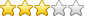Knowledgebase# CONVERTING GAS UNITS TO KILOWATT-HOURS (KWH)

Solution

Below is a step by step process for calculating gas kWh:

#### Calculation for imperial gas meters

1) Number of units used                                                                                    100

2) Convert from imperial to metric – multiply number of units used by 2.83 (fixed) 100’s cu ft x 2.83.   10’s cu ft x 0.283.   cu ft x 0.0283                                                                                                       283

3) Multiply by volume correction factor (1.022640)                                       289.407

4) Multiply by calorific value (39.3)                                                           11373.699

5) Divide by kWh conversion factor (3.6- Fixed)                                         3159.361

6) Multiply by read factor                                                                         3159.361

Note that the correction factor changes

Note that the calorific value changes

Read factor to be determined by supplier / meter details

#### Calculation for metric gas meters

1) Number of units used                                                                                        100

2) Multiply by volume correction factor (1.022640)                                         102.264

3) Multiply by calorific value (39.3)                                                             4018.975

4) Divide by kWh conversion factor (3.6 -Fixed)                                          1116.382

Note that the correction factor changes

Note that the calorific value changes

Read factor to be determined by supplier / meter details

 Article ID: 67 Category: Technical Articles Date added: 2014-07-31 12:41:46 Rating (Votes):(18)